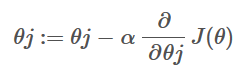# Can someone explain me the difference between a cost function and the gradient descent equation in logistic regression?

+1 vote
1 view

I'm going through the ML-Class on Coursera on Logistic Regression and also the Manning Book Machine Learning in Action. I'm trying to learn by implementing everything in Python.

I'm not able to understand the difference between the cost function and the gradient. There are examples on the net where people compute the cost function and then there are places where they don't and just go with the gradient descent function

w: = w - (alpha) * (delta)w * f(w)

What is the difference between the two if any?

+1 vote
by (33.2k points)
edited by

Cost Function for logistic regression:

• We use a cost function called Cross-Entropy, also known as Log Loss.

• Cross-entropy loss can be divided into two separate cost functions: one for y=1 and one for y=0

For example:

def cost_function(features, labels, weights):

observations = len(labels)

predictions = predict(features, weights)

#Take the error when label=1

class1_cost = -labels*np.log(predictions)

#Take the error when label=0

class2_cost = (1-labels)*np.log(1-predictions)

#Take the sum of both costs

cost = class1_cost + class2_cost

#Take the average cost

cost = cost.sum() / observations

return cost

This code will return the cost of the features.

• Gradient descent is by far the most popular optimization strategy, used in Machine Learning and Deep Learning at the moment

• It is used while training your model, can be combined with every algorithm, and is easy to understand and implement.

• To minimize our cost, we use Gradient Descent.For example:

def update_weights(features, labels, weights, lr):

N = len(features)

#Get Predictions

predictions = predict(features, weights)

gradient = np.dot(features.T,  predictions - labels)

#Take the average cost derivative for each feature

# - Multiply the gradient by our learning rate

#5 - Subtract from our weights to minimize cost

return weights